# NCERT Solutions for Class 6 Maths Algebra Chapter 11:Exercise 11.4,11,5

In this page we have NCERT Solutions for Class 6 Maths Algebra Chapter 11 Exercise 11.4,11,5. Hope you like them and do not forget to like , social share and comment at the end of the page.
Topics of Use of variables in common rules,Expressions with variables,Using expressions practically,What is an equation?,Solution of an equation is deleted from this chapter as per rationalized syllabus. So you may ignore the exercise 11.4,11.5

## Exercise 11.4

Question 1
(a) Take Sarita’s present age to be y years
(i) What will be her age 5 years from now?
(ii) What was her age 3 years back?
(iii) Sarita’s grandfather is 6 times her age. What is the age of her grandfather?
(iv) Grandmother is 2 years younger than grandfather. What is grandmother’s age?
(v) Sarita’s father’s age is 5 years more than 3 times Sarita’s age. What is her father’s age?
(b) The length of a rectangular hall is 4 meters less than 3 times the breadth of the hall. What is the length, if the breadth is b meters?
(c) A rectangular box has height h cm. Its length is 5 times the height and breadth is 10 cm less than the length. Express the length and the breadth of the box in terms of the height.
(d) Meena, Beena and Leena are climbing the steps to the hill top. Meena is at step s, Beena is 8 steps ahead and Leena 7 steps behind. Where are Beena and Meena? The total number of steps to the hill top is 10 less than 4 times what Meena has reached. Express the total number of steps using s.
(e) A bus travels at v km per hour. It is going from Daspur to Beespur. After the bus has travelled 5 hours, Beespur is still 20 km away. What is the distance from Daspur to Beespur? Express it using v.
(a) Given Sarita’s present age to be y years
(i) Her age 5 years from now = her present age + 5 = y + 5 years.
(ii) Her age 3 years back = her present age – 3 years = y – 3 years
(iii) Sarita’s grandfather age = 6 times her age = 6y years
(iv) Grandmother’s age = Grandfather’s age – 2 = 6y – 2 years
(v) Sarita’s father’s age is 5 years more than 3 times Sarita’s age = 5 + 3y
(b) breadth of the rectangular hall = b meters
length of the hall = 4 meters less than 3 times the breadth = 3b – 4 meters
(c) height of the rectangular box = h cm
length = 5 times the height = 5h cm
breadth = 10 cm less than the length = 5h – 10 cm
(d) Step at which Meena is = s
Step at which Beena is = Meena’s step + 8 steps = s + 8
Step at which Leena is = Meena’s step – 7 = s – 7
Total number of steps = 4s – 10
(e) Distance travelled by the bus in 1 hour = v km
Distance travelled in 5 hours = 5v km
So, total distance from Daspur to Beespur = Distance travelled in 5 hours + 20 = 5v + 20 km

Question 2
Change the following statements using expressions into statements in ordinary language.
(For example, Given Salim scores r runs in a cricket match, Nalin scores
(r + 15) runs. In ordinary language − Nalin scores 15 runs more than Salim.)
(a) A note book costs Rs p. A book costs Rs 3 p.
(b) Tony puts q marbles on the table. He has 8 q marbles in his box.
(c) Our class has n students. The school has 20 n students.
(d) Jaggu is z years old. His uncle is 4 z years old and his aunt is (4z − 3) years old.
(e) In an arrangement of dots there are r rows. Each row contains 5 dots.
Here is my attempt to say it in ordinary statement
(a) The cost of a book is three times the cost of a notebook.
(b) Tony has 8 times the number of marbles in his box than on the table.
(c) Total number of students in the class is 20 times that of our class.
(d) Jaggu’s uncle is 4 times the age of Jaggu and his aunt is 3 less than 4 times the age of Jaggu.
(e) The total number of dots is 5 times the number of rows

Question 3
(a) Given Munnu’s age to be x years, can you guess what (x − 2) may show?
(Hint: Think of Mannu’s younger brother.)
Can you guess what (x + 4) may show? What (3x + 7) may show?
(b) Given Sara’s age today to be y years. Think of her age in the future or in the past.
What will the following expression indicate? $y+7,y-3,y+4 \frac{1}{2} ,y-2 \frac {1}{2}$
(c) Given n students in the class like football, what may 2n show? What may  show? (Hint: Think of games other than football).
(a) If Munnu’s age is x years, then (x – 2) may show the age is 2 years younger than Munnu.
(x + 4) shows that the age is 4 years older than Munnu and 3x + 7 shows that the age considered is 7 more than thrice the age of Munnu.
(b) Sara’s present age = y years
y + 7 represents her future age, i.e., her age after 7 years.
y – 3 represents her past age, i.e. her age 3 years ago.
y + 4½ represents her future age, i.e. her age after 4½ years
y - 2½ represents her past age, i.e. her age 2½ years ago.
(c) 2n represents twice the number of students who like football. n/2 represents half the number of students who like football.
It may represent the number of students who like cricket, or basketball etc.

## Exercise 11.5

Question 1
State which of the following are equations (with a variable). Give reason for your answer. Identify the variable from the equations with a variable.
(a) $17 =x+ 7$
(b) $(t- 7) > 5$
(c) $\frac {4}{2}=2$
(d) $(7 \times 3) - 19 = 8$
(e) $5 \times 4 - 8 = 2x$
(f) $x- 2 = 0$
(g) $2m < 30$
(h) $2n+ 1 = 11$
(i) $7 = (11 \times 5) - (12 \times 4)$
(j) $7 = (11 \times 2) +p$
(k) $20 = 5y$
(l) $\frac {3q}{2} < 5$
(m) $z+ 12 > 24$
(n) $20 - (10 - 5) = 3 \times 5$
(o) $7 -x= 5$
Equation has = sign
While inequation as > or < sign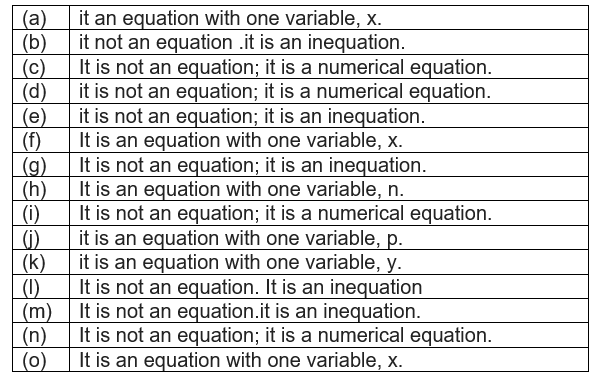Question 2
Complete the entries in the third column of the table.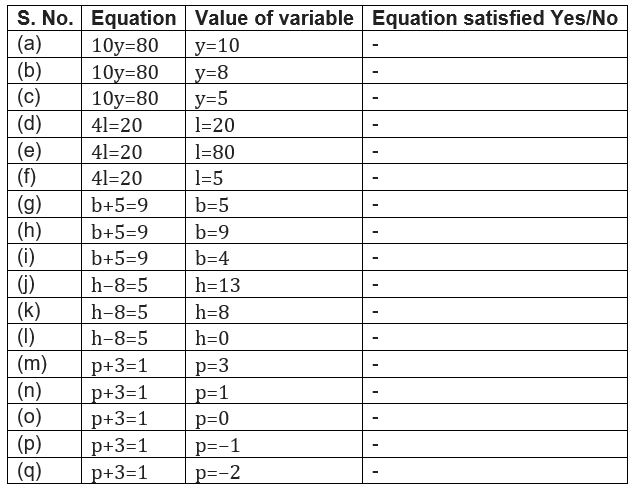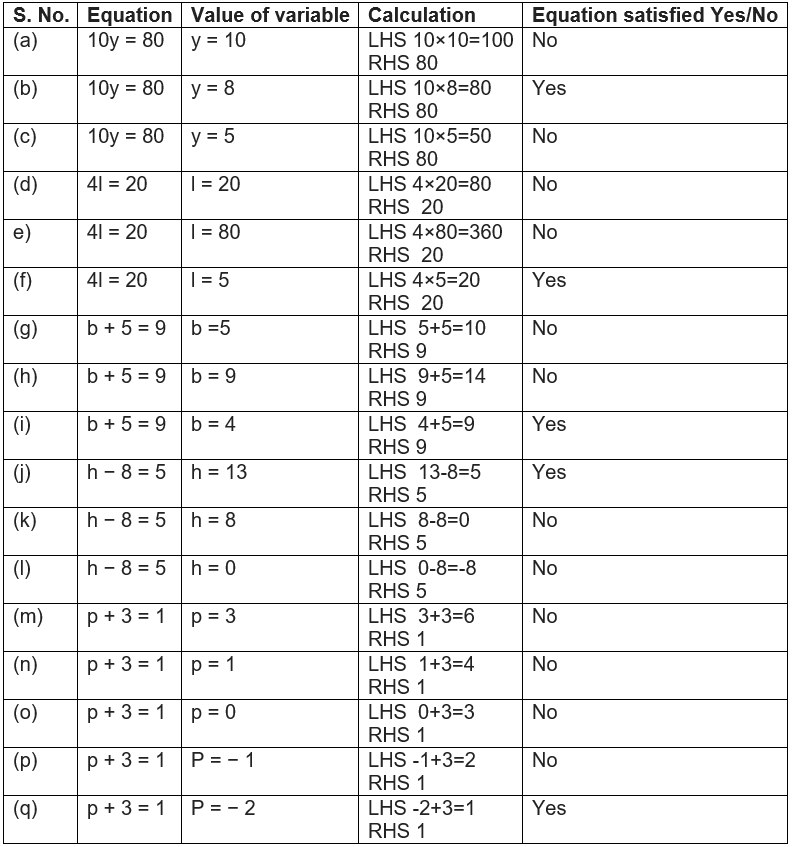Question 3
Pick out the solution from the values given in the bracket next to each equation. Show that the other values do not satisfy the equation.
(a) $5m= 60$ (10, 5, 12, 15)
(b) $n+ 12 = 20$ (12, 8, 20, 0)
(c) $p - 5 = 5$ (0, 10, 5 − 5)
(d) $\frac {q}{2}=7$ (7, 2, 10, 14)
(e) $r - 4 = 0$ (4, − 4, 8, 0)
(f) $x+ 4 = 2$ (− 2, 0, 2, 4)
(a) $5m= 60$
Let's try the equation of each given value of m
For m = 10, 5m = 5(10) = 50
For m = 5, 5m = 5(5) = 25
For m = 12, 5m = 5(12) = 60
For m = 15, 5m = 5(15) = 75
So, we see that m = 12 satisfy the equation and is the solution.
(b)$n+ 12 = 20$
Let's try the equation of each given value of n
For n = 12, n + 12 = 12 + 12 = 24
For n = 8, n + 12 = 8 + 12 = 20
For n = 20, n + 12 = 20 + 12 = 32
For n = 0, n + 12 = 0 + 12 = 12
So, we see that n = 8 satisfy the equation and is the solution.
(c)$p - 5 = 5$
Let's try the equation of each given value of p
For p = 0, p – 5 = 0 – 5 = -5
For p = 10, p – 5 = 10 – 5 = 5
For p = 5, p – 5 = 5 – 5 = 0
For p = -5, p – 5 = -5 – 5 = -10
So, we see that p = 10 satisfy the equation and is the solution.
(d)$\frac {q}{2}=7$
Let's try the equation of each given value of q
For q = 7, q/2 = 7/2
For q = 2, q/2 = 2/2 = 1
For q = 10, q/2 = 10/2 = 5
For q = 14, q/2 = 14/2 = 7
So, we see that q = 14 satisfy the equation and is the solution.
(e)$r - 4 = 0$
Let's try the equation of each given value of r
For r = 4, r – 4 = 4 – 4 = 0
For r = -4, r – 4 = -4 – 4 = -8
For r = 8, r – 4 = 8 – 4 = 4
For r = 0, r – 4 = 0 – 4 = -4
So, we see that r = 4 satisfy the equation and is the solution.
(f)$x+ 4 = 2$
Let's try the equation of each given value of x
For x = -2, x + 4 = -2 + 4 = 2
For x = 0, x + 4 = 0 + 4 = 4
For x = 2, x + 4 = 2 + 4 = 6
For x = 4, x + 4 = 4 + 4 = 8
So, we see that x = -2 satisfy the equation and is the solution.

Question 4
(a) Complete the table and by inspection of the table, find the solution to the equation m + 10 = 16.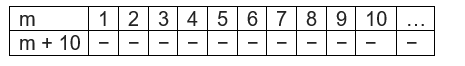(b) Complete the table and by inspection of the table, find the solution to the equation 5t = 35.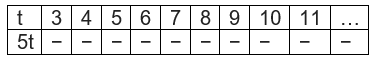(c) Complete the table and find the solution of the equation z/3 = 4 using the table.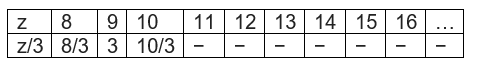(d) Complete the table and find the solution to the equation m − 7 = 3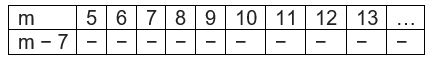(a)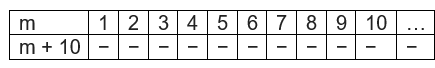From the table, solution for m + 10 = 16 is m=6
(b)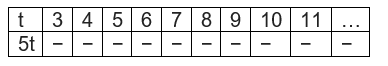From the table, solution for 5t = 35 is t=7
(c)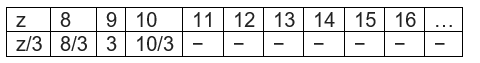From the table, solution for z/3 = 4 is z=12
(d)From the table, solution for equation m − 7 = 3 is m=10
Question 5
Solve the following riddles, you may yourself construct such riddles.
Who am I?
(i) Go round a square ,Counting every corner,Thrice and no more! ,Add the count to me, To get exactly thirty-four!
(ii) For each day of the week,Make an up count from me, If you make no mistake, You will get twenty-three!
(iii) I am a special number,Take away from me a six! ,A whole cricket team ,You will still be able to fix!
(iv) Tell me who I am ,I shall give a pretty clue! ,You will get me back ,If you take me out of twenty-two!
i) A square has 4 sides.
“Counting every corner Thrice and no more!”
Implies we go around the square thrice, i.e. 12 times.
“Add the count to me to get exactly thirty-four!”
That is, when 12 is added to a number
Let us assume the number as y, we get 34.
12 + y = 34
y = 34 – 12 = 22
So, 22 is the number.
ii)
If 23 is the number for Sunday,
Then counting up, Saturday is 22, Friday is 21, Thursday is 20, Wednesday is 19, Tuesday is 18, Monday is 17 and Sunday is 16.
Therefore, the number considered is 16.
iii)
Let the number be x.
If we take away 6 from x, we get a cricket team. [A cricket team has 11 players].
x – 6 = 11
x = 11 + 6 = 17
The special number is 17.
iv)
Let the number be x.
22 – x = x
22 = x + x = 2x
x = 22/2 = 11
The number is 11.### Practice Question

Question 1 What is $\frac {1}{2} + \frac {3}{4}$ ?
A)$\frac {5}{4}$
B)$\frac {1}{4}$
C)$1$
D)$\frac {4}{5}$
Question 2 Pinhole camera produces an ?
A)An erect and small image
B)an Inverted and small image
C)An inverted and enlarged image
D)None of the above